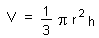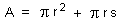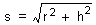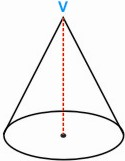HOME MATH DICTIONARY DOWNLOAD FEEDBACK DISCLAIMER
 Question: What is Right Cone or Pyramid ? Answer: A right cone or pyramid is one where the vertex V is right above the center of its base. When this is not the case it is called oblique, in which the axis does not pass perpendicularly through the centre of the base. The formula for volume of a right cone is :where, r = radius and h = height The formula for area of a right cone is :where, r = radius and